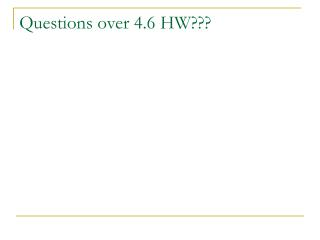DownloadDownload PresentationQuestions over 4.6 HW???

# Questions over 4.6 HW???

Télécharger la présentation## Questions over 4.6 HW???

- - - - - - - - - - - - - - - - - - - - - - - - - - - E N D - - - - - - - - - - - - - - - - - - - - - - - - - - -
##### Presentation Transcript

1. Questions over 4.6 HW???

2. 4.7 (Green)Solve Exponential and Logarithmic Equations No School: Monday Logarithms Test: 1/21/10 (Thursday)

3. Vocabulary: • Exponential equations are equations in which variable expressions occur as exponents. • Logarithmic equations are equations that involve logarithms of variable expressions.

4. Vocabulary: • Property of Equality for Exponential Equations: • If b is a positive number other than 1, then bx = by if and only if x = y. • Property of Equality for Logarithmic Equations: • If b, x, and y are positive numbers with b = 1, then logbx = logby if and only if x = y.

5. Example 1: • Solve 8x = 4x + 1

6. You Try: • Solve 53x + 1 = 25x + 1

7. Example 2: • Solve 8x = 23

8. You Try: • Solve 43x = 9

9. Example 3: • Solve log8(x + 6) = log8(4 – x)

10. You Try: • Solve • log7(x + 2) = log7(2 – 3x) • ln(4 – 5x) = ln(x + 10)

11. Example 4: • Find the solution(s) of log x + log(x + 3) = 1 You need to check for extraneous solutions….fakers!

12. You Try: • Solve • log6 x + log6(x – 5) = 2 • 2 ln x = ln(2x – 3) + ln(x – 2)

13. Homework: • Pg. 161 #1-41odd You need to practice! Your FIRST test (55% of your grade) is next THURSDAY!!!!